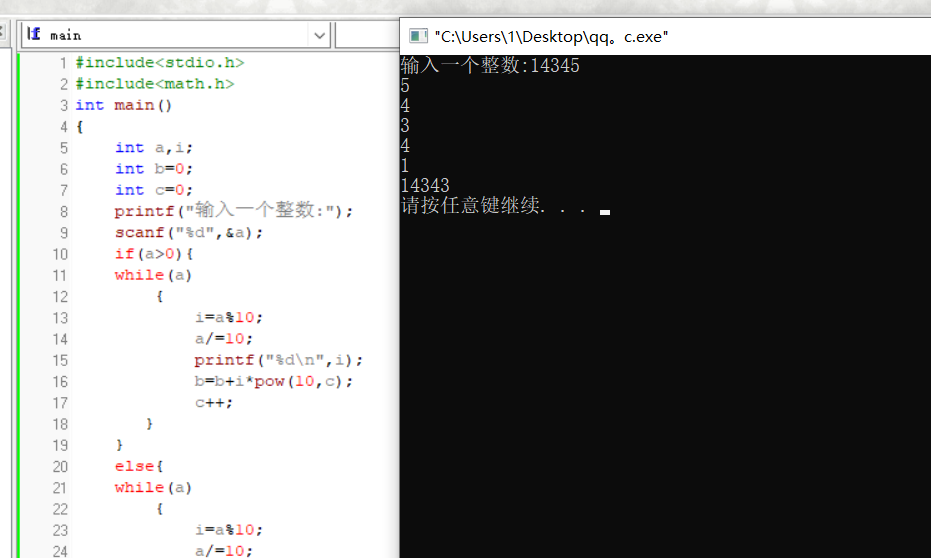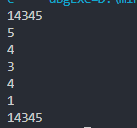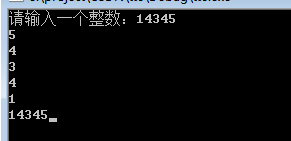2021-11-25 23:02

# 输入一个整数，将它的个十百千输出，再和成这个数，可是为什么输出的会小一点• 写回答
• 好问题 提建议
• 关注问题
• 收藏
• 邀请回答

#### 2条回答默认 最新

•你这部分代码没有问题，是不是下面的代码有问题，把代码发完整看看
或者参考

``````#include<stdio.h>
#include<math.h>

int main()
{
int a,i;
int b = 0;
int c = 0;
scanf("%d", &a);
while (a)
{
i = a%10;
a /= 10;
printf("%d\n", i);
b = b+i*pow(10,c);
c++;
}
printf("%d\n", b);

return 0;
}
``````编辑记录

评论
解决 无用
打赏 举报
•这些代码看着没什么问题，你把代码贴全。参考如下代码：

代码：

``````#include <stdio.h>
#include <math.h>

int main()
{
int a,i;
int b=0;
int c =0;
printf("请输入一个整数：");
scanf("%d",&a);
if (a>0)
{
while(a)
{
i=a%10;
a/=10;
printf("%d\n",i);
b = b+ i*pow(10.0,c);
c++;
}
}else
{
while(a)
{
i=a%10;
a/=10;
printf("%d\n",i);
b = b+i*pow(10.0,c);
c++;
}
}
printf("%d",b);
return 0;
}

``````

编辑记录

评论
解决 无用
打赏 举报